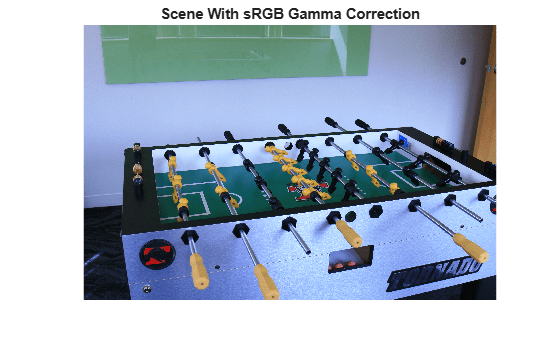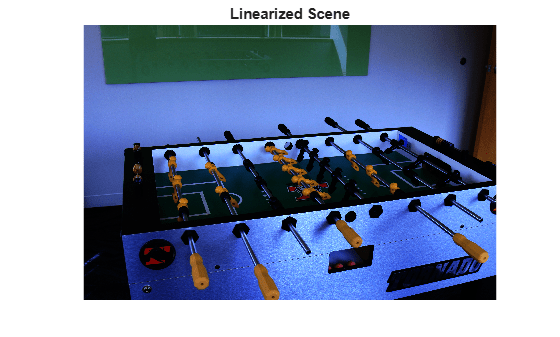# rgb2lin

Linearize gamma-corrected RGB values

## Syntax

``B = rgb2lin(A)``
``B = rgb2lin(A,Name,Value)``

## Description

````B = rgb2lin(A)` undoes the gamma correction of the sRGB values in image `A` so that `B` contains linear RGB values.```

example

````B = rgb2lin(A,Name,Value)` undoes gamma correction using name-value arguments to control additional options.```

## Examples

collapse all

Open an image. The JPEG file format saves images in the gamma-corrected sRGB color space.

`A = imread("foosball.jpg");`

Display the image.

```imshow(A) title("Scene With sRGB Gamma Correction")```Undo the gamma correction and linearize the image by using the `rgb2lin` function. Optionally, specify the data type of the linearized values.

`B = rgb2lin(A,OutputType="double");`

Display the linearized image. Shadows in the linearized image are darker than in the original image, as expected.

```imshow(B) title("Linearized Scene")```## Input Arguments

collapse all

Gamma-corrected RGB color values, specified as a numeric array in one of the following formats.

• c-by-3 colormap. Each row specifies one RGB color value.

• m-by-n-by-3 image

• m-by-n-by-3-by-p stack of images

Data Types: `single` | `double` | `uint8` | `uint16`

### Name-Value Arguments

Specify optional pairs of arguments as `Name1=Value1,...,NameN=ValueN`, where `Name` is the argument name and `Value` is the corresponding value. Name-value arguments must appear after other arguments, but the order of the pairs does not matter.

Example: `B = rgb2lin(I,ColorSpace="adobe-rgb-1998")` linearizes the gamma-corrected image, `I`, according to the Adobe RGB (1998) standard.

Before R2021a, use commas to separate each name and value, and enclose `Name` in quotes.

Example: `B = rgb2lin(I,"ColorSpace","adobe-rgb-1998")` linearizes the gamma-corrected image, `I`, according to the Adobe RGB (1998) standard.

Color space of the input image, specified as `"srgb"`, `"adobe-rgb-1998"`, or `"prophoto-rgb"`.

Data Types: `char` | `string`

Data type of the output RGB values, specified as `"double"`, `"single"`, `"uint8"`, or `"uint16"`. By default, the output data type is the same as the data type of `A`.

Data Types: `char` | `string`

## Output Arguments

collapse all

Linearized RGB color values, returned as a numeric array of the same size as the input `A`.

## Algorithms

collapse all

### Linearization Using the sRGB Standard

sRGB tristimulus values are linearized using this parametric curve:

f(u) = -f(-u),                u < 0

f(u) = cu,                0 ≤ u < d

f(u) = (au + b)ɣ,      ud,

where u represents a color value with these parameters:

a = 1/1.055

b = 0.055/1.055

c = 1/12.92

d = 0.04045

ɣ = 2.4

### Linearization Using the Adobe RGB (1998) Standard

Adobe RGB (1998) tristimulus values are linearized using a simple power function :

v = uɣ,

with

ɣ = 2.19921875

### Linearization Using the ProPhoto (ROMM RGB) Standard

ProPhoto (ROMM RGB) tristimulus values are linearized using this parametric curve :

f(u) = 0,             u < 0

f(u) = u/16,             0 ≤ u < 16*Et

f(u) = u1.8 + b,      16*Etu < 1,

f(u) = 1,             u ≥ 1

with

Et = 1/512

 Ebner, Marc. "Gamma Correction." Color Constancy. Chichester, West Sussex: John Wiley & Sons, 2007.

 Adobe Systems Incorporated. "Inverting the color component transfer function." Adobe RGB (1998) Color Image Encoding. Section 4.3.5.2, May 2005, p.12.

 ISO 22028-2:2013 Photography and graphic technology — Extended colour encodings for digital image storage, manipulation and interchange — Part 2: Reference output medium metric RGB colour image encoding (ROMM RGB). https://www.iso.org/standard/56591.html